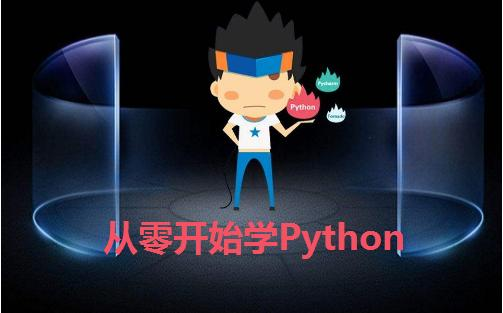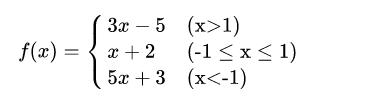# 从零开始学Python：05-分支结构if语句的使用

"""

Version: 0.1
Author: 骆昊
"""
print('身份验证成功!')
else:
print('身份验证失败!')"""

Version: 0.1
Author: 骆昊
"""
x = float(input('x = '))
if x > 1:
y = 3 * x - 5
elif x >= -1:
y = x + 2
else:
y = 5 * x + 3
print(f'f({x}) = {y}')

"""

Version: 0.1
Author: 骆昊
"""
x = float(input('x = '))
if x > 1:
y = 3 * x - 5
else:
if x >= -1:
y = x + 2
else:
y = 5 * x + 3
print(f'f({x}) = {y}')

"""

Version: 0.1
Author: 骆昊
"""
value = float(input('请输入长度: '))
unit = input('请输入单位: ')
if unit == 'in' or unit == '英寸':
print('%f英寸 = %f厘米' % (value, value * 2.54))
elif unit == 'cm' or unit == '厘米':
print('%f厘米 = %f英寸' % (value, value / 2.54))
else:
print('请输入有效的单位')

"""

Version: 0.1
Author: 骆昊
"""
score = float(input('请输入成绩: '))
if score >= 90:
elif score >= 80:
elif score >= 70:
elif score >= 60:
else:
print('对应的等级是:', grade)

"""

Version: 0.1
Author: 骆昊
"""
a = float(input('a = '))
b = float(input('b = '))
c = float(input('c = '))
if a + b > c and a + c > b and b + c > a:
peri = a + b + c
print(f'周长: {peri}')
half = peri / 2
area = (half * (half - a) * (half - b) * (half - c)) ** 0.5
print(f'面积: {area}')
else:
print('不能构成三角形')04-262万+

#### 最新java学习资料汇总（学习路线+视频教程+网盘下载）

04-081万+

#### WEB学习路线2020完整版（附视频教程+学习资料）

04-067397

#### 最新UI设计师教程（学习路线+课程大纲+视频教程+面试题+学习工具）

04-061万+

#### 2020最新java学习教程（学习路线+课程大纲+视频教程+面试题+学习工具）

02-091万+

#### Java视频教程从入门到精通(2020完整版)

04-161万+

#### python机器学习视频教程百度网盘下载？

07-0270

#### 《MySQL数据库》MySql简介、下载与安装

07-0255

#### 《MySQL数据库》MySql连接方式和数据类型

07-0280

#### 《MySQL数据库》SQL简介、语法格式

07-0255

#### 《MYSQL数据库》TCL语言和DCL语言

07-0246

#### 《MySQL数据库》常用函数整理

07-0156

#### javaSE中的==和equals的联系与区别

07-0181

#### 从零开始学Python：第七课-关于分支和循环结构的应用

07-0156

#### Hdfs安装模式之完全分布式集群

07-0152

07-0172

07-0164

#### HDFS设计思想和相关概念

07-0152

#### 《MySQL数据库》数据库相关概念及历史发展

06-3072

#### 从零开始学Python：第十课-函数和字符串的应用

06-3074

#### 从零开始学Python：第九课-常用数据结构之字符串点击重新获取扫码支付1.余额是钱包充值的虚拟货币，按照1:1的比例进行支付金额的抵扣。
2.余额无法直接购买下载，可以购买VIP、C币套餐、付费专栏及课程。余额充值